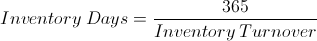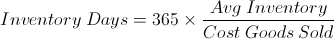# Days in InventoryThe formula to calculate days in inventory is the number of days in the period divided by the inventory turnover ratio. This formula is used to determine how quickly a company is converting their inventory into sales. A slower turnaround on sales may be a warning sign that there are problems internally, such as brand image or the product, or externally, such as an industry downturn or the overall economy.

The numerator of the days in inventory formula is shown at the top of this page as 365 to denote 365 days in a year. However, it is important to match the period in the numerator with the period for the inventory turnover used. For example, suppose that a company is calculating the days in inventory held based on a inventory turnover of 4.32 for one year. This can be divided into 365 days of the year for an average days in inventory of 84.49. If the same company has an inventory turnover of 2.31 for 180 days, the average days in inventory would be 77.92.

## How is the Days in Inventory Formula Derived?

To understand the days in inventory held formula, one must look at the inventory turnover formula used in the denominator.It is important to remember that the average inventory for the period is used. From here, the days in inventory formula can be rewritten as the numerator multiplied by the inverse of the denominator.The 2nd portion of this formula is essentially the % of goods left to be sold, in terms of cost. This % of goods left to be sold can be used to estimate the % of time it is held prior to sale. The % of time products are held prior to sale can be converted into actual days by multiplying by 365 days in a year, or in a period.

## Considerations of the Days in Inventory Formula

As with any financial formula, knowing how to compute days in inventory does not imply the ability to apply deductive reasoning to understand the formula in practice. One issue to consider with the days in inventory formula is how cost of goods sold and inventory is calculated. Is one company using LIFO to calculate inventory and another company using the weighted average method?

Another issue to consider is companies with seasonal sales. If a company sales primarily at the beginning of the year, perhaps their inventory will be extraordinarily high at the end of the year to prepare for the following month.

It is important to work towards holding all things constant when comparing one company to the next, or even one year to the next.

New to Finance?